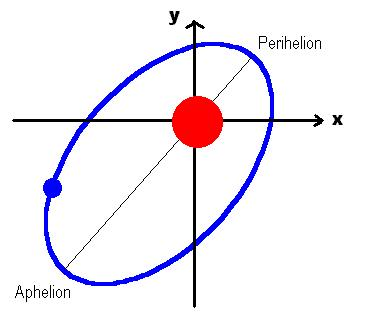# LAB 10

## Objectives

• Structured Problem-Solving
• Representing Functions as Arrays
• Searching and Sorting Parallel Arrays

## Planetary Motion

A scientist is tracking the position of a planet relative to a coordinate system whose origin is at the Sun and whose axes point at fixed stars. It is known that the orbit is an ellipse with the Sun at its focus. The major axis of the ellipse intersects with its circumference at two points known as the perihelion and aphelion:It is also known that at the perihelion, the distance between the planet and the Sun is shortest and its orbital speed is largest. Whereas at at the aphelion, the distance between the planet and the Sun is largest and its orbital speed is smallest.

Beginning one day, to be called day #0, and about every week thereafter, the scientist records the day number (0, 1, 2, ...) and the x-y coordinates of the planet on a piece of paper. After several years, it was decided to automate the analysis of the recorded data and, hence, these pieces of paper were collected and entered, albeit not in order, into a text file named: orbit.txt (you can download this file by right-clicking its name and choosing to save it in the same directory in which you will write the program for this Lab). Each record in the file contains three `real*8`, space-delimitted numbers: the day number (not sorted), the x-coordinate, and the y-coordinate (measured in kilometers). For array declaration purposes, you can assume that the file has less than a thousand records.

We need to analyze this data to determine certain orbital attributes. To that end, we follow the structured approach and divide the problem into subproblems and then write the following main program:

```
program orbit
implicit none
real*8 dayAR(1000), xAR(1000), yAR(1000)
real*8 min, max
integer*4 lengthAR, maxSize /1000/, permute(1000)

call loadFile(dayAR, xAR, yAR, lengthAR, maxSize)
call sortMe(dayAR, xAR, yAR, permute, lengthAR)

call orbital(dayAR, xAR, yAR, lengthAR, minMaxOrb, min, max)

call showGeo(minMaxOrb)

end ```
Here are descriptions (and hints) for each subprogram:
• `loadFile`
Open the file and read its contents into the three arrays, computing the actual number of elements as you read. The populated arrays and the size are returned to the caller. Note that `maxSize` is an input parameter (sent by the caller to the sub) to enable array declaration, whereas `lengthAR` is an output parameter (returned from the sub to the caller) to let the caller know the actual size of the arrays. The reading loop is terminated upon encountering end-of-file; i.e. when the integer*4 variable set by `IOSTAT = variable` becomes non-zero (the 3rd argument of the `read` statement). This can be implemented in ~20 lines.

• `sortMe`
Sort the three arrays as parallel (i.e. synchronized, corresponding) arrays. This is best done by using the `dpSort` routine in `SLATEC` to generate a sorted permutation (`permute`) of it, and then using the `dpPerm` routine to physically re-arrange the three arrays based on that permutation. This can be implemented in ~10 lines.

• `radial`
This sub analyzes the data and returns the x-y coordinates of the perihelion and the aphelion. It locates the perihelion (aphelion) by finding the point at which the planet was closest to (farthest from) the Sun. To facilitate parameter transfer, it returns these four real*8 coordinates in one array of 4 elements. This can be implemented in ~30 lines.

• `orbital`
This sub analyzes the data and returns the x-y coordinates of the perihelion and the aphelion. It locates the perihelion (aphelion) by finding the point at which the planet's orbital speed was largest (smallest). To facilitate parameter transfer, it returns these four real*8 numbers in one array of 4 elements. It also returns the computed smallest and largest speeds. This can be implemented in ~30 lines.

• `showGeo`
This is an output-only subprogram used to verify that the computed points are indeed what we expect them to be. This sub outputs the angles, in degrees, of the line joining the Sun and the perihelion, the Sun and the aphelion, and the perihelion with the aphelion. It also outputs the semi-major axis length (in millions of km) assuming the two helions and the Sun are indeed on a straight line. All figures are printed with two decimals only. Something like this (your numbers must be different):
```
Angles of A, B, and of AB:    56.99  -120.28    58.85
Semi major axis (in M.km):    799.11 ```
where A refers to the first point and B to the second, as received from the caller in the 4-element array. Use the `atan2` function to compute the angle given the x,y coordinate of a point; viz. atan2(y,x), and then convert to degrees. Note that main invokes this sub twice, once sending radial data and once orbital data. The two results should be close but may not be identical.

• `showPeriod`
This is also an output only sub. It outputs the min / max orbital speeds (in km/s) and an estimate of the planet's orbital period (in Earth years). All figures are printed with two decimals only. Hint: for period computation purposes, assume the orbit is apprximately a circle. The output should look like this:
```      Minimum orbital speed (in km/sec) =     xx.xx
Maximum orbital speed (in km/sec) =     xx.xx
Planet's period  (in Earth years) =     xx.xx
```

### Deliverables

1. Printed program
2. A log showing the program's output (a single run).
3. A brief explanation of how you computed the planet's period.
4. This is optional: a quantitative explanation of the difference between the two methods of computing the semi-major axis length.

Fall2002/HR